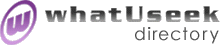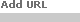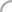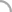SearchThe Directory The Webfor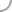Differential_EquationsNew! Submit a site

Categories:
 Courses (3)Journals (4)Events (41)whatUseek Collection Sites (submit a site ):

Give your site great placement in this category in as little as two business days!

whatUseek Directory Site Listings:

Analytic Solution for the Burgers Equation - Provides the general analytic solution for the Burgers equation in the form of a 4-D commutative hypercomplex function. The solution exhibits the main dynamic features in a Burgers medium: propagation of disturbances, shock waves, propagating state change fronts, and solitons. A page is included to explain the hypercomplex mathematics.

Bifurcations, Equilibria, and Phase Lines: Modern Topics in Differential Equation Courses - Online course material

Computational PDEs Unit - School of Computing, University of Leeds. Research details, publications, software and resources.

Differential Equations in Banach Algebras - Fuchsian Singularities of Linear Ordinary Differential Equations in Banach Algebras. By Gerald Albrecht in Wuppertal.

Differential Equations in Industry and Commerce - EU TMR network coordinated at Oxford Centre for Industrial and Applied Mathematics.

GetDP (a General environment for the treatment of Discrete Problems) - A scientific software environment for the numerical solution of integro-differential equations, open to the coupling of physical problems (electromagnetic, acoustic, thermal, mechanical, ...) as well as of numerical methods (finite element methods, boundary element and integral methods, ...).

Introduction to ODEs with Mathematica

Kevin Brown's Calculus and DiffEq Notes - Many topics on differential equations are covered including the Laplace Transform.

MGNet - Information related to multigrid, multilevel, multiscale, aggregation, defect correction, and domain decomposition methods.

Math Unit III: More on the derivative and differential equations - Exact definition of derivation and calculating the relationship of derivatives of related functions.

Nonlinear Differential Equations at Glasgow - The site describes research activities of the differential equations group in the mathematics department at the university of Glasgow, UK, and provides some resources of a general nature.

Numerical Methods for Partial Differential Equations - Methods such as finite differences, finite elements, fast Fourier transforms, Monte-Carlo and Lagrangian schemes are discussed in 1D to solve a variety of problems including the advection, diffusion, Black-Scholes, Burger, Korteweg-DeVries and the Schroedinger equations.

ODEcalc an ODE calculator! - An Ordinary Differential Equation (ODE) Calculator! State your equation and boundary or initial value conditions and it solves your problem. Plots solution, y, and derivative, ydot, versus x. Solves nth order ODE as IVP & BVP.

Osaka University - PDE-Analysis research group. Organises the East Asia Symposium on PDE.

PDEase2D 3.0 Main Page - PDEase solves partial differential equations numerically by finite element analysis solving problems in heat transfer, reaction diffusion, solid mechanics, fluid mechanics, electromagnetics, groundwater flow, quantum mechanics and more.

PRIDE - Products by Rapid Integrated Detailed Engineering. An application of PDEs in engineering design.

Software for Differential Equations

Stripf's Homepage - A Java Applet to illustrate and solve initial value problems. Uses different numerical methods (e.g. Runge-Kutta) that can be compared to each other.

Table of Laplace Transforms - This page contains an extensive table of Laplace transforms. Laplace transforms are used to solve certain differential equations.

[ 1 2 ]Help build the largest human-edited directory on the web.About   Help   Content Filter   Terms   Privacy Policy© 2018 whatUseek